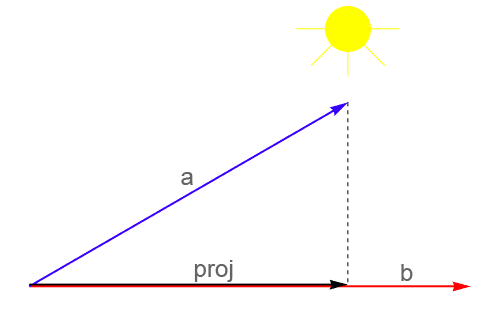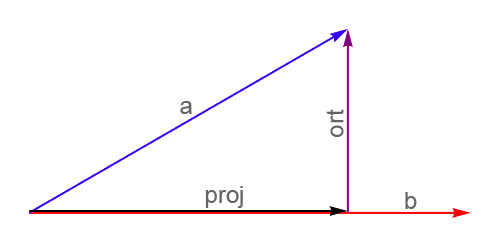# Vector Projection Calculator

By Miroslav Jerkovic, PhD
Last updated: Jun 26, 2020

This vector projection calculator finds the projection of one vector onto the other. We start with two vectors, a and b, which are not on the same line. Imagine a light source above the vectors. Now, think of the vector projection of a onto b as the shadow that vector a projects on the direction of vector b.

This is why this type of vector is known as a projection vector (marked as proj in the image):Imagining vector projection as the shadow

Another, more technical, way of intuiting the idea of vector projection is to ask a question: how much of the vector a goes in the same direction of vector b?

## Where does the vector projection formula come from

Here is the vector projection formula our calculator uses to find the projection of vector a onto the vector b:

`p = (a·b / b·b) * b`.

The formula utilises the dot product, a·b, of the vectors, also called the scalar product. But, where did this vector projection formula come from?

On the image above, there is a hidden vector. This is the vector orthogonal to vector b, sometimes also called the rejection vector (denoted by ort in the image):Vector projection and rejection

Here is a step by step procedure on how to get to the vector projection formula:

1. Decompose the vector a into a sum of the projection and the rejection vectors: `a = proj + ort`

2. Express proj as `proj = a - ort`

3. Also, since proj is parallel to b, we can write it as `proj = C * b`, where C is some unknown factor we want to determine

4. Now we have `C * b = a - ort`

5. Calculate the dot product of the both sides of this equation with the vector b: `C * b·b = a·b - ort·b`

6. Since the vector ort is orthogonal to the vector b, their dot product is zero and we have `C * b·b = a·b`

7. Finally, we have `C = a·b / b·b`.

When you insert this expression for C into `proj = C * b`, you get the formula:

`p = (a·b / b·b) * b`!

Since this formula uses the dot product, which can be defined for vectors of any integer dimension, this formula covers vectors of any dimensionality. Its practical applications are for 2-D and 3-D vectors, which is why our calculator is designed for vectors with two or three components.

Also, please be aware that this formula is sometimes called the orthogonal projection formula. If we followed this terminology, we'd have to call our calculator the orthogonal projection calculator. There isn't a big difference either way, it'll work the same :)!

## How to use the vector projection calculator

Let a and b be `a = 2i -3j + 5k` and `b = 3i + 6j - 4k`. From this point on, we will write these 3-D vectors in their component form

`a = [2, -3, 5]` and `b = [3, 6, -4]`.

Use the vector projection calculator to find out the projection of vector a onto vector b. The result we get is

`proj = [-1.5738, -3.1475, 2.0984]`.

If you want to calculate the projection by hand, use the vector projection formula `p = (a·b / b·b) * b` and follow this step by step procedure:

1. Calculate the dot product of vectors a and b: `a·b = 2*3 + (-3)*6 + 5*(-4) = -32`

2. Calculate the dot product of vector b with itself: `b·b = 3*3 + 6*6 + (-4)*(-4) = 61`

3. Insert these two dot products into the vector projection formula to get `proj = (-32/61) * [3, 6, -4] = [-96/61, -192/61, 128/61]`.

This result, when expressed decimally, equals the result obtained by this calculator.

Maybe you wondered what happens when a is orthogonal to b; what does the projection proj look like? In this case, the shadow of vector a that is perpendicular to b should be non-existent, so we should get the null vector.

This is reflected in the vector projection formula: if a and b are orthogonal, their dot product is zero: `a·b = 0`. Now we have:

`proj = (0 / b·b) * b = 0 * b = 0`.

Explore this special case is shown in the following example:

`a = [2, 6, -3]` and `b = [6, 4, 12]`.

You shouldn't be surprised when `[0, 0, 0]` pops out, since this is the correct result!

## Vector projection in physics – playing with forces

One of the most straightforward, and maybe the most interesting, uses of vector projection formula comes from the physics of forces and their decomposition.

Imagine a cart rolling down the slope of the hill. What is the force one should use to counterbalance the force of the cart? Of course, this counterforce is dependent on the weight of the cart: the more massive the cart, the harder it will be to stop. Also, the slope of the hill itself plays a role - the steeper the hill is, the more force you will need to stop the cart. Here is an image of the situation:Cart on the slope

Here α is the angle of the hill's slope relative to the ground, and F the force of gravity between the cart and the Earth. Notice that vector F is perpendicular to the ground, not to the hill's slope itself.

To find the force needed to counterbalance gravity's action on the cart, we should calculate the projection of the force vector F along the direction of the hill. Let's say that `F = 400 N` (which corresponds to the cart with a mass of around `m = 40 kg`) and that the hill's slope is `α = 45°`.

The question is, how do you find a vector along the direction of the hill's slope, the one to project the force vector F on? We can use any vector that has the same direction as the hill's slope, so the most convenient one will be the unit vector `u = [cos 45°, sin 45°]`, marked as the blue vector on the above image. Also, for the force vector F we take `F = [0, -400]`. The negative sign here means that force F is directed downwards.

Use the vector projection calculator and choose to work with vectors in two dimensions, since we're dealing with a two-dimensional problem. So, the vector a will be equal to the force vector F;

`a = [0, -400]`,

and the vector b is obtained from the vector u, using the sine and cosine calculators to find the values for `sin 45°` and `cos 45°`:

`b = [0.70710678, 0.70710678]`.

Working with these numbers, we get the projection vector:

`proj = [-200, -200]`.

Since we want to know the magnitude of the counterbalancing force, we can use the vector magnitude calculator to find the magnitude of proj. It is equal to `282.84 N`.

If you play a bit with the hill's slope, i.e. with the angle α, you will see that the less narrow the hill, the smaller force yo will need, while in the case of a larger α - a steeper hill - you will need a larger required reactive force.

## Other real-world applications of vector projection formula

Besides physics and the problem of finding a component of force along a given direction, vector projection is used in the important statistical problem of linear regression, as well as in Principal Component Analysis, one of the most used methods in data science. Here, one is confronted with a task of representing many, possibly high-dimensional, data points within a space of lower dimensionality, in such a way that there is as minimal information loss as possible.

Miroslav Jerkovic, PhDDimensions
3D
Vector a
x
y
z
Vector b
x
y
z
Projection of a onto b
x
y
z
Projection factor
People also viewed…

### Black Friday

How to get best deals on Black Friday? The struggle is real, let us help you with this Black Friday calculator!